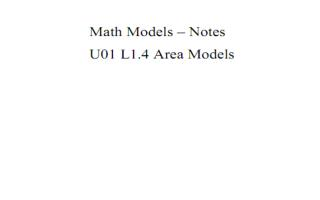DownloadDownload Presentationtotal

# total

Télécharger la présentation## total

- - - - - - - - - - - - - - - - - - - - - - - - - - - E N D - - - - - - - - - - - - - - - - - - - - - - - - - - -
##### Presentation Transcript

1. total desired circles polygons

2. 3 2 1 1 1 = 8 6 4 2 3

3. A circle has 360o total degrees. 180 - 45 Half a circle has 180o degrees. 135o 120o 180 - 60 135 3 = 8 360 60 1 = 360 6 120 1 = 360 3 45 1 = 360 8

4. total Area = 10 x 10 = 100 b2 1 trapezoid Area = ( )5(3 + 7) = 25 2 h 25 1 = P(falling inside the trapezoid) = b1 4 100 Area of a trapezoid formula 1 A = ( )h(b1 + b2) 2

5. 1 triangle area = (8.66)(90) = 389.7 in2 2 circle area = л(8.66)2 = 235.6 in2 235.6 P(hitting circle) = 60.5 % = = 0.605 389.7 Area of a triangle Area of a circle 1 A = aP A = л r2 2 a = apothem (distance from the center to midpoint of a regular polygon)